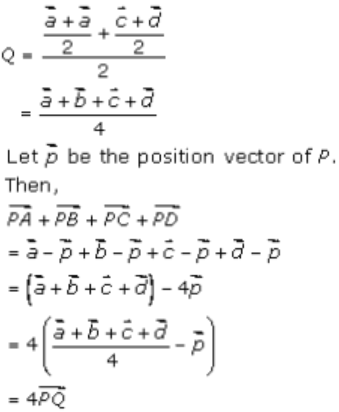# RD Sharma Solutions Class 12 Algebra Of Vectors Exercise 23.4

RD Sharma Solutions for Class 12 Maths Chapter 23 Algebra of Vectors Exercise 23.4 are given here to help students prepare for their exams at ease. Practising the textbook problems will primarily benefit the students to analyse their level of preparation and also assess their conceptual knowledge. Students can now easily download the RD Sharma Class 12 Solutions PDF of this exercise for free, from the link given below.

## Download PDF of Rd Sharma Solution for Class 12 Maths Chapter 23 Exercise 4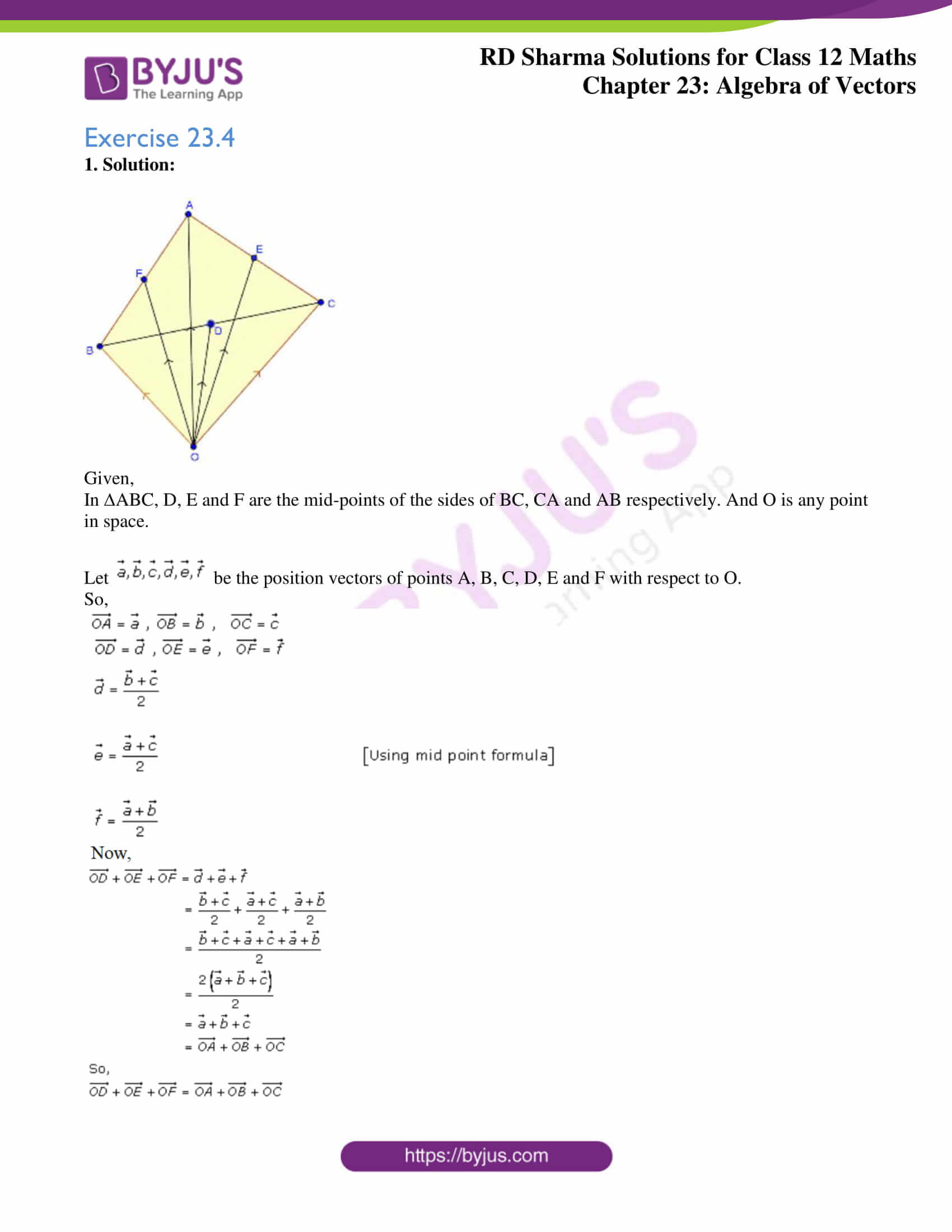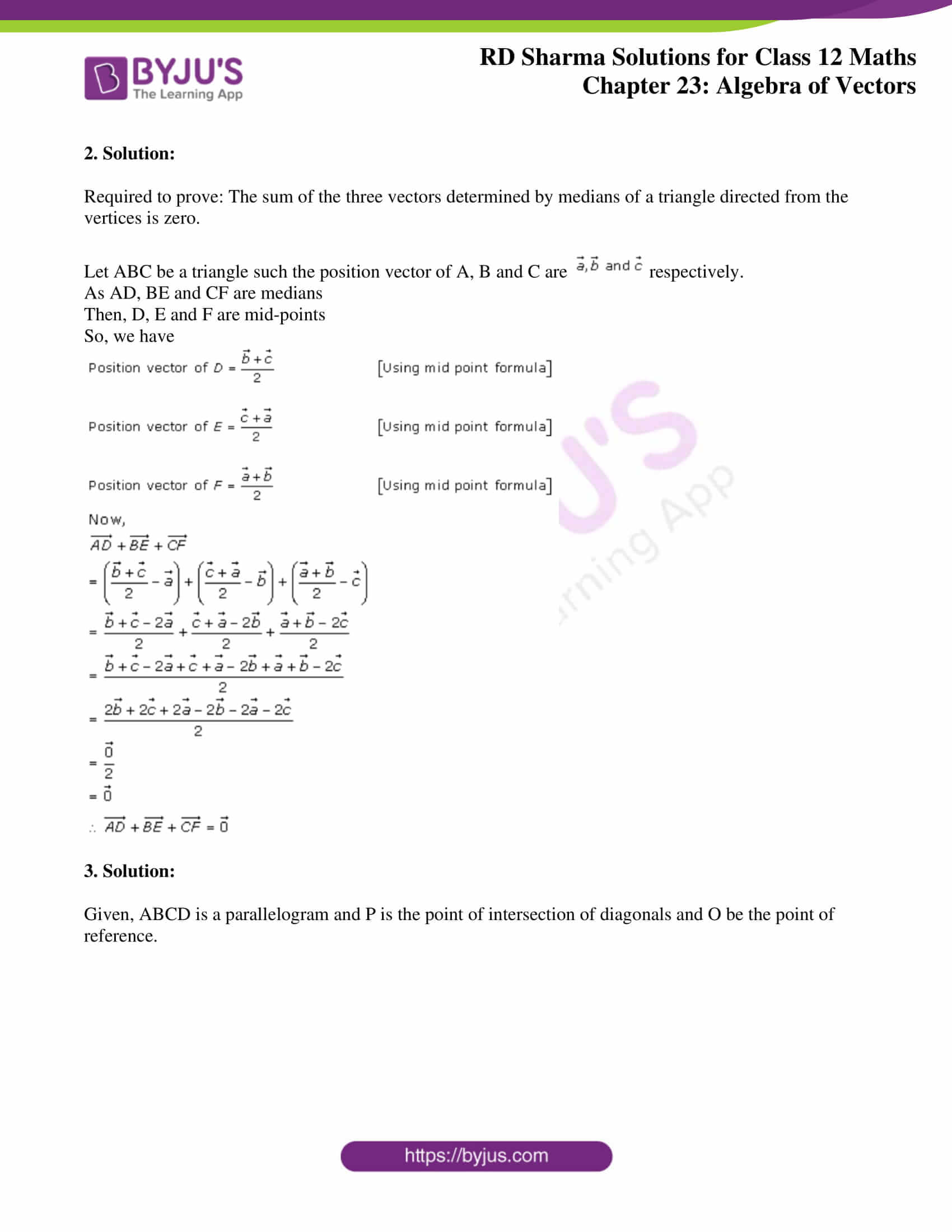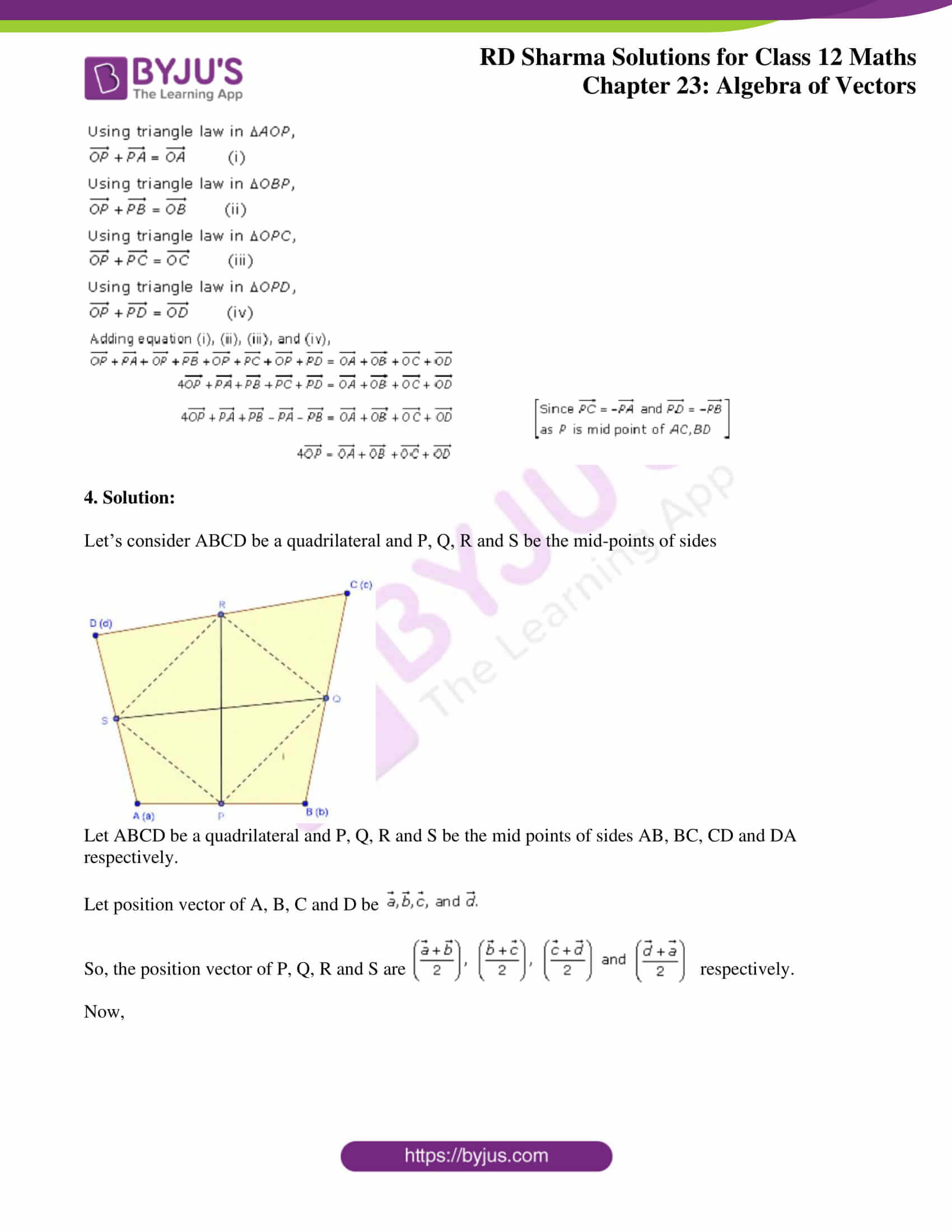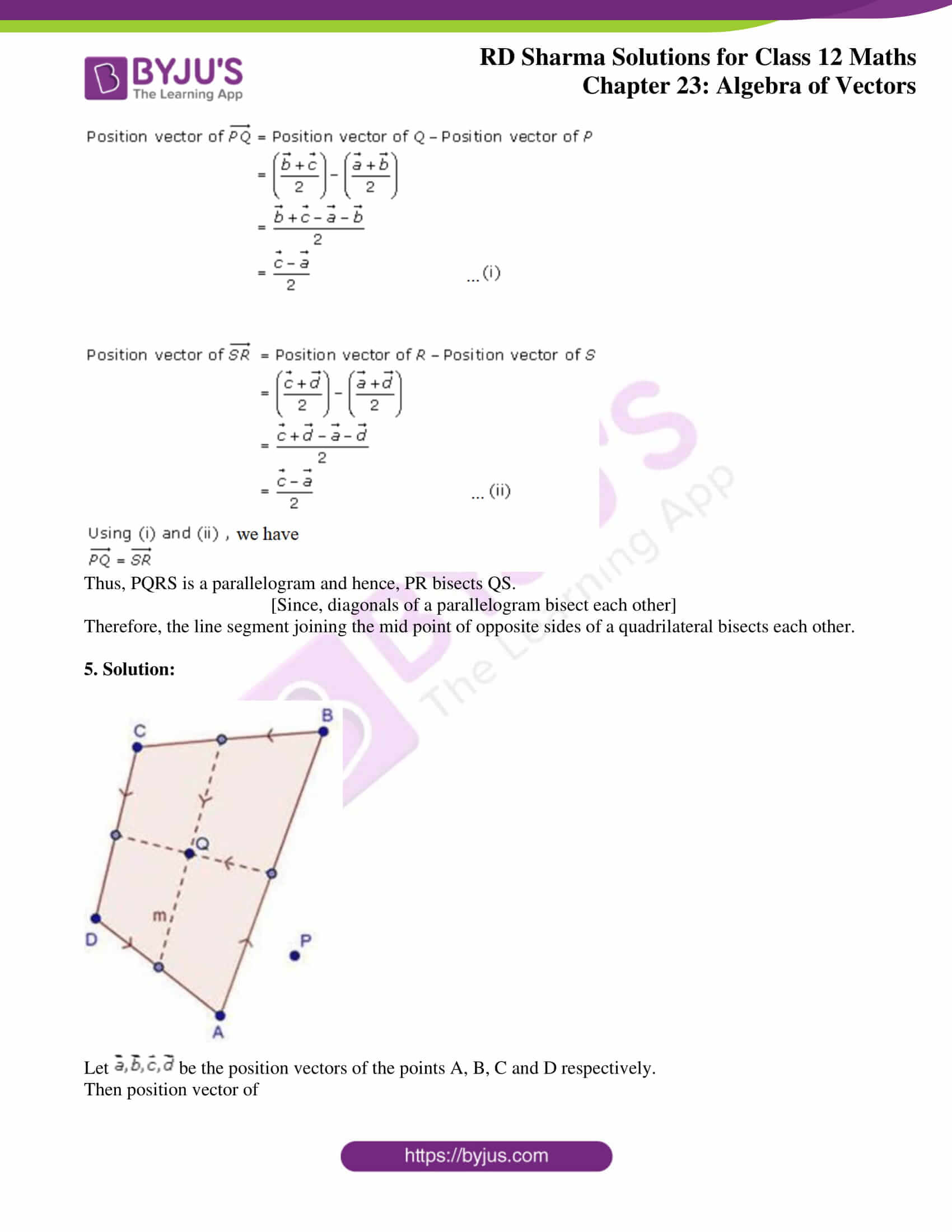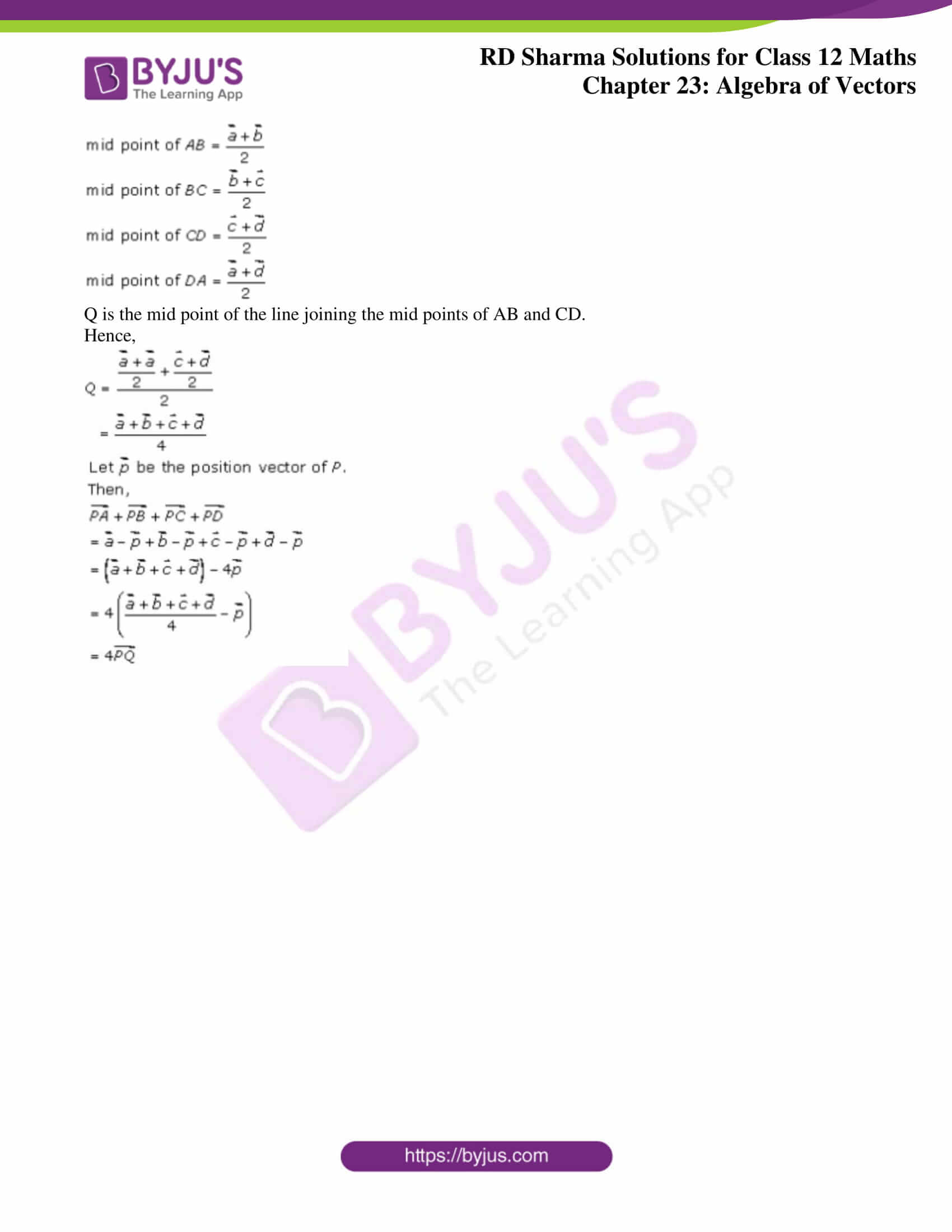### Access Answers for Rd Sharma Solution Class 12 Maths Chapter 23 Exercise 4

Exercise 23.4

1. Solution: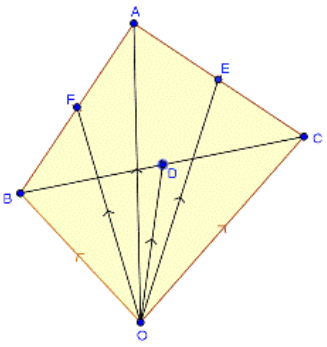Given,

In ∆ABC, D, E and F are the mid-points of the sides of BC, CA and AB respectively. And O is any point in space.

Let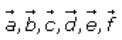be the position vectors of points A, B, C, D, E and F with respect to O.

So,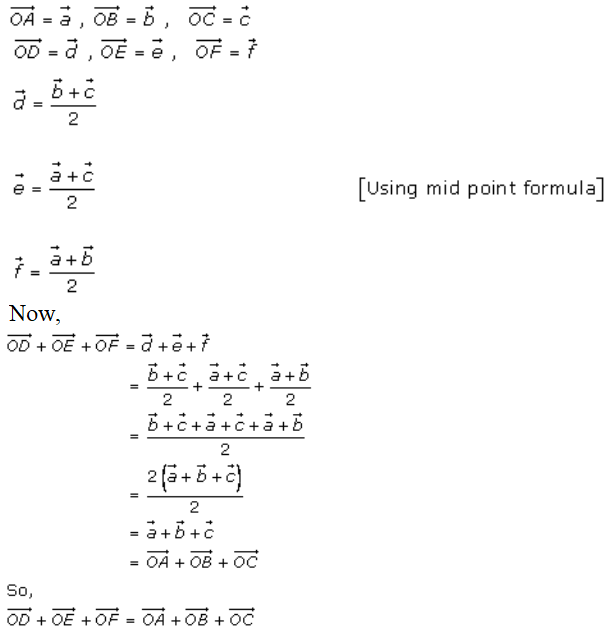2. Solution:

Required to prove: The sum of the three vectors determined by medians of a triangle directed from the vertices is zero.

Let ABC be a triangle such the position vector of A, B and C are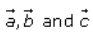respectively.

As AD, BE and CF are medians

Then, D, E and F are mid-points

So, we have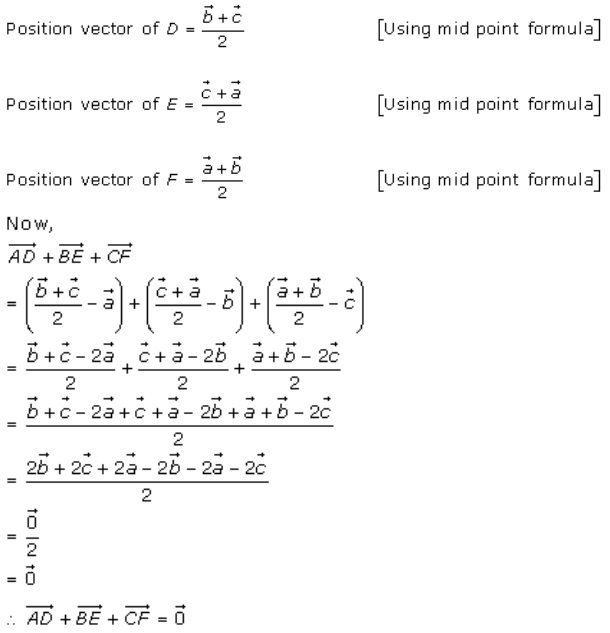3. Solution:

Given, ABCD is a parallelogram and P is the point of intersection of diagonals and O be the point of reference.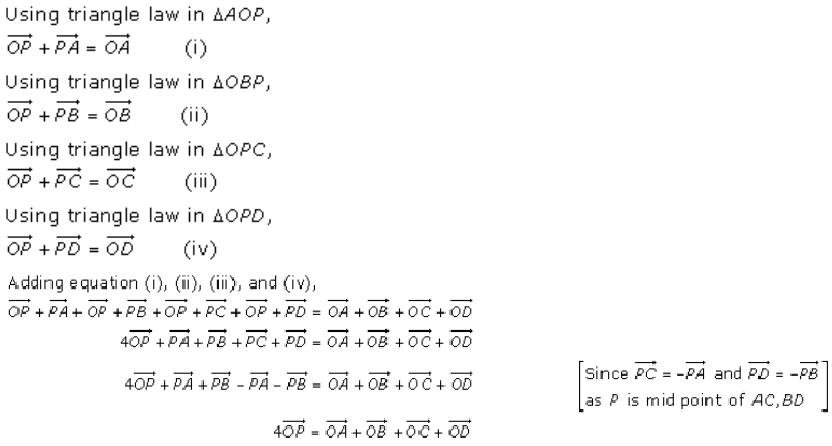4. Solution:

Let’s consider ABCD be a quadrilateral and P, Q, R and S be the mid-points of sides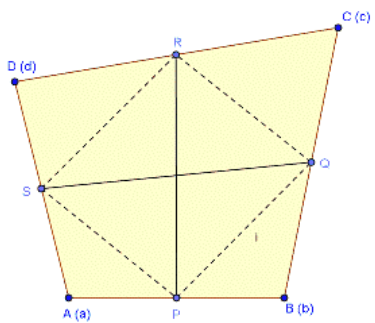Let ABCD be a quadrilateral and P, Q, R and S be the mid points of sides AB, BC, CD and DA respectively.

Let position vector of A, B, C and D be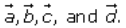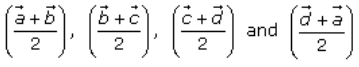So, the position vector of P, Q, R and S are respectively.

Now,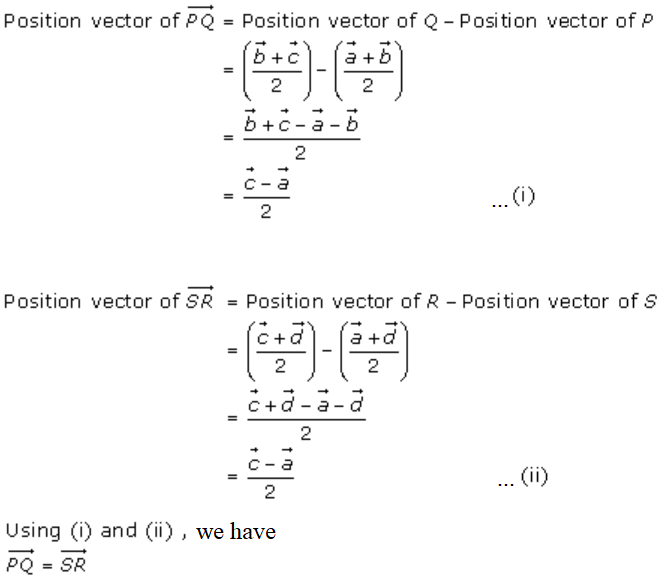Thus, PQRS is a parallelogram and hence, PR bisects QS.

[Since, diagonals of a parallelogram bisect each other]

Therefore, the line segment joining the mid point of opposite sides of a quadrilateral bisects each other.

5. Solution: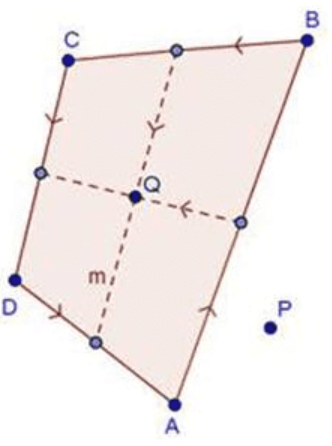Let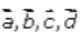be the position vectors of the points A, B, C and D respectively.

Then position vector of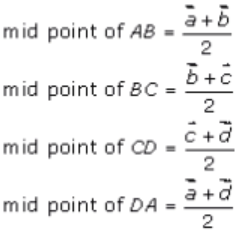Q is the mid point of the line joining the mid points of AB and CD.

Hence,